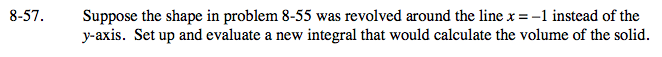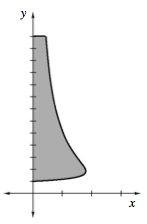### Home > CALC > Chapter 8 > Lesson 8.1.6 > Problem8-57

8-57.

Suppose the shape in problem 8-55 was revolved around the line x = −1 instead of the y-axis. Set up and evaluate a new integral that would calculate the volume of the solid. Homework Help ✎Like problem 8-55, the rotating radius will be horizontal (because the axis of rotation is vertical). Consequently, the bounds of integration will be y-values: from y = 1 to y = 13. Both the bounds and the integrand will be written in terms of y.

Unlike problem 8-55, there will be a cylindrical hole in the center of this 3D figure. This hole has a radius of 1.

Unlike problem 8-55, the outer function is no longer f(y). It is 1 unit longer than f(y).